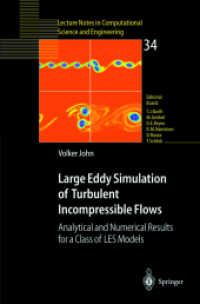### Large Eddy Simulation of Turbulent Incompressible Flows : Analytical and Numerical Results for a Class of LES Models (Lecture Notes in Computational Science and Engineering Vol.34) （2003. 300 p.）

#### ドイツ（DK）から取り寄せる

• 在庫がございません。海外の書籍取次会社を通じて出版社等からお取り寄せいたします。
通常6～9週間ほどで発送の見込みですが、商品によってはさらに時間がかかることもございます。
重要ご説明事項
1. 納期遅延や、ご入手不能となる場合がございます。
2. 複数冊ご注文の場合、分割発送となる場合がございます。
3. 美品のご指定は承りかねます。

#### アメリカ（US）から取り寄せる

• 提携先の海外書籍取次会社に在庫がございます。通常3週間で発送いたします。
重要ご説明事項
1. 納期遅延や、ご入手不能となる場合が若干ございます。
2. 複数冊ご注文の場合、分割発送となる場合がございます。
3. 美品のご指定は承りかねます。
• 【重要：入荷遅延について】
ウクライナ情勢悪化・新型コロナウィルス感染拡大により、洋書・洋古書の入荷が不安定になっています。詳しくはこちらをご確認ください。
海外からのお取り寄せの場合、弊社サイト内で表示している標準的な納期よりもお届けまでに日数がかかる見込みでございます。
申し訳ございませんが、あらかじめご了承くださいますようお願い申し上げます。
• ◆画像の表紙や帯等は実物とは異なる場合があります。
• 製本 Paperback:紙装版/ペーパーバック版／ページ数 300 p.
• 商品コード 9783540406433

### Full Description

Large eddy simulation (LES) seeks to simulate the large structures of a turbulent flow. This is the first monograph which considers LES from a mathematical point of view. It concentrates on LES models for which mathematical and numerical analysis is already available and on related LES models. Most of the available analysis is given in detail, the implementation of the LES models into a finite element code is described, the efficient solution of the discrete systems is discussed and numerical studies with the considered LES models are presented.

### Contents

1 Introduction.- 1.1 Short Remarks on the Nature and Importance of Turbulent Flows.- 1.2 Remarks on the Direct Numerical Simulation (DNS) and the k — ? Model.- 1.3 Large Eddy Simulation (LES).- 1.4 Contents of this Monograph.- 2 Mathematical Tools and Basic Notations.- 2.1 Function Spaces.- 2.2 Some Tools from Analysis and Functional Analysis.- 2.3 Convolution and Fourier Transform.- 2.4 Notations for Matrix-Vector Operations.- 3 The Space Averaged Navier-Stokes Equations and the Commutation Error.- 3.1 The Incompressible Navier-Stokes Equations.- 3.2 The Space Averaged Navier-Stokes Equations in the Case ? = ?d.- 3.3 The Space Averaged Navier-Stokes Equations in a Bounded Domain.- 3.4 The Gaussian Filter.- 3.5 Error Estimate of the Commutation Error Term in the Lp (?d) Norm.- 3.6 Error Estimate of the Commutation Error Term in the H-1 (?) Norm.- 3.7 Error Estimate for a Weak Form of the Commutation Error.- 4 LES Models Which are Based on Approximations in Wave Number Space.- 4.1 Eddy Viscosity Models.- 4.1.1 The Smagorinsky Model.- 4.1.2 The Dynamic Subgrid Scale Model.- 4.2 Modelling of the Large Scale and Cross Terms.- 4.2.1 The Taylor LES Model.- 4.2.2 The Second Order Rational LES Model.- 4.2.3 The Fourth Order Rational LES Model.- 4.3 Models for the Subgrid Scale Term.- 4.3.1 The Second Order Fourier Transform Approach.- 4.3.2 The Fourth Order Rational LES Model.- 4.3.3 The Smagorinsky Model.- 4.3.4 Models Proposed by Iliescu and Layton.- 5 The Variational Formulation of the LES Models.- 5.1 The Weak Formulation of the Equations.- 5.2 Boundary Conditions for the LES Models.- 5.2.1 Dirichlet Boundary Condition.- 5.2.2 Outflow or Do-Nothing Boundary Condition.- 5.2.3 Free Slip Boundary Condition.- 5.2.4 Slip With Linear Friction and No Penetration Boundary Condition.- 5.2.5 Slip With Linear Friction and Penetration With Resistance Boundary Condition.- 5.2.6 Periodic Boundary Condition.- 5.3 Function Spaces for the LES Models.- 6 Existence and Uniqueness of Solutions of the LES Models.- 6.1 The Smagorinsky Model.- 6.1.1 A priori error estimates.- 6.1.2 The Galerkin Method.- 6.2 The Taylor LES Model.- 6.3 The Rational LES Model.- 7 Discretisation of the LES Models.- 7.1 Discretisation in Time by the Crank-Nicolson or the Fractional-Step ?-Scheme.- 7.2 The Variational Formulation and the Linearisation of the Time-Discrete Problem.- 7.3 The Discretisation in Space.- 7.4 Inf-Sup Stable Pairs of Finite Element Spaces.- 7.5 The Upwind Stabilisation for Lowest Order Non-Conforming Finite Elements.- 7.6 The Implementation of the Slip With Friction and Penetration With Resistance Boundary Condition.- 7.7 The Discretisation of the Auxiliary Problem in the Rational LES Model.- 7.8 The Computation of the Convolution in the Rational LES Model.- 7.9 The Evaluation of Integrals, Numerical Quadrature.- 8 Error Analysis of Finite Element Discretisations of the LES Models.- 8.1 The Smagorinsky Model.- 8.1.1 The Variational Formulation and Stability Estimates.- 8.1.2 Goal of the Error Analysis and Outline of the Proof.- 8.1.3 The Error Equation.- 8.1.4 The Case ?w ? L3 (0, T; L3 (?)) and a0 (?).- 8.1.5 The Case ?w ? L3 (0, T; L3 (?)) and a0 (?).- 8.1.6 The Case ?w ? L2 (0, T; L? (?)) and a0 (?).- 8.1.7 Failures of the Present Analysis in Other Interesting Cases.- 8.1.8 A Numerical Example.- 8.2 The Taylor LES Model.- 9 The Solution of the Linear Systems.- 9.1 The Fixed Point Iteration for the Solution of Linear Systems.- 9.2 Flexible GMRES (FGMRES) With Restart.- 9.3 The Coupled Multigrid Method.- 9.3.1 The Transfer Between the Levels of the Multigrid Hierarchy.- 9.3.2 The Vanka Smoothers.- 9.3.3 The Standard Multigrid Method and the Multiple Discretisation Multilevel Method.- 9.3.4 Schematic Overview and Parameters.- 9.4 The Solution of the Auxiliary Problem in the Rational LES Model.- 10 A Numerical Study of a Necessary Condition for the Acceptability of LES Models.- 10.1 The Flow Through a Channel.- 10.2 The Failure of the Taylor LES Model.- 10.3 The Rational LES Model.- 10.3.1 Computations With the Smagorinsky Subgrid Scale Model.- 10.3.2 Computations With the Iliescu-Layton Subgrid Scale Model.- 10.3.3 Computations Without Model for the Subgrid Scale Term.- 10.4 Summary.- 11 A Numerical Study of the Approximation of Space Averaged Flow Fields by the Considered LES Models.- 11.1 A Mixing Layer Problem in Two Dimensions.- 11.1.1 The Definition of the Problem and the Setup of the Numerical Tests.- 11.1.2 The Smagorinsky Model and the Rational LES Model With Smagorinsky Subgrid Scale Term.- 11.1.3 The Rational LES Model With Iliescu-Layton Subgrid Scale Term.- 11.1.4 The Rational LES Model Without Model for the Subgrid Scale Term.- 11.1.5 A Comparison of the Smagorinsky Subgrid Scale Term and the Iliescu-Layton Subgrid Scale Term.- 11.2 A Mixing Layer Problem in Three Dimensions.- 11.2.1 The Definition of the Problem and the Setup of the Numerical Tests.- 11.2.2 Evaluation of the Numerical Results.- 12 Problems for Further Investigations.- 13 Notations.- References.